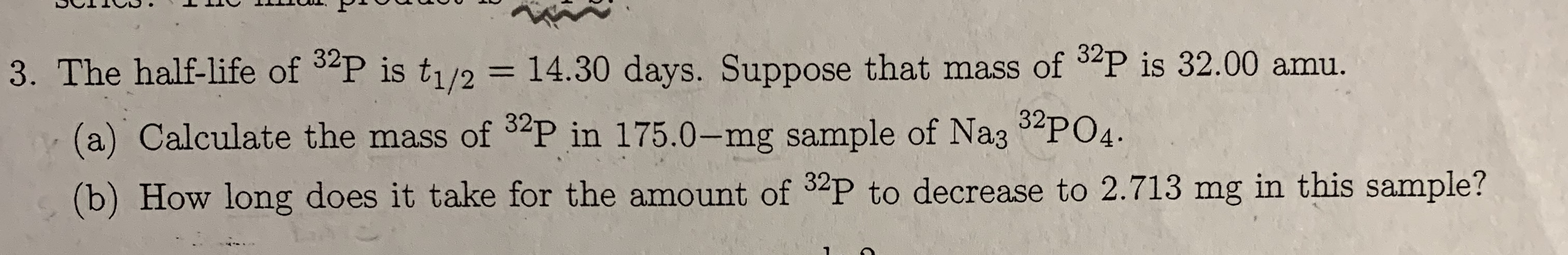# 3. The half-life of 3P is t1/2 = 14.30 days. Suppose that mass of P is 32.00 amu. (a) Calculate the mass of 2P in 175.0-mg sample of Na35PO4 (b) How long does it take for the amount of 32P to decrease to 2.713 mg in this sample?

Questionhelp_outlineImage Transcriptionclose3. The half-life of 3P is t1/2 = 14.30 days. Suppose that mass of P is 32.00 amu. (a) Calculate the mass of 2P in 175.0-mg sample of Na35PO4 (b) How long does it take for the amount of 32P to decrease to 2.713 mg in this sample? fullscreen

### Want to see this answer and more?

Experts are waiting 24/7 to provide step-by-step solutions in as fast as 30 minutes!*

*Response times vary by subject and question complexity. Median response time is 34 minutes and may be longer for new subjects.
Tagged in
ScienceChemistry

### Chemical Kinetics